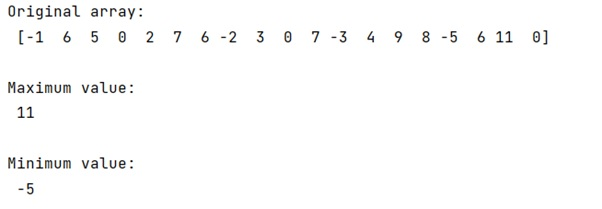# Find the min/max excluding zeros in a numpy array (or a tuple)

Learn, how to find the min/max excluding zeros in a numpy array (or a tuple) in Python?
Submitted by Pranit Sharma, on February 08, 2023

NumPy is an abbreviated form of Numerical Python. It is used for different types of scientific operations in python. Numpy is a vast library in python which is used for almost every kind of scientific or mathematical operation. It is itself an array which is a collection of various methods and functions for processing the arrays.

## Finding the min/max excluding zeros in a numpy array

Suppose that we are given a NumPy array that may contain some negative values, 0, and some positive values and we need to find the maximum and minimum value in this array after excluding the zero.

For this purpose, we will first return all the non-zero elements of the numpy array using numpy.nonzero(arr) command and then we will apply numpy.min() and numpy.max() methods on this.

Let us understand with the help of an example,

## Python code to find the min/max excluding zeros in a numpy array (or a tuple)

```# Import numpy
import math

import numpy as np

# Creating a numpy array
arr = np.array([-1,6,5,0,2,7,6,-2,3,0,7,-3,4,9,8,-5,6,11,0])

# Display original array
print("Original array:\n",arr,"\n")

# Finding max and min
min = np.min(arr[np.nonzero(arr)])
max = np.max(arr[np.nonzero(arr)])

# Display maximum
print("Maximum value:\n",max,"\n")

# Display minimum
print("Minimum value:\n",min,"\n")
```

Output: